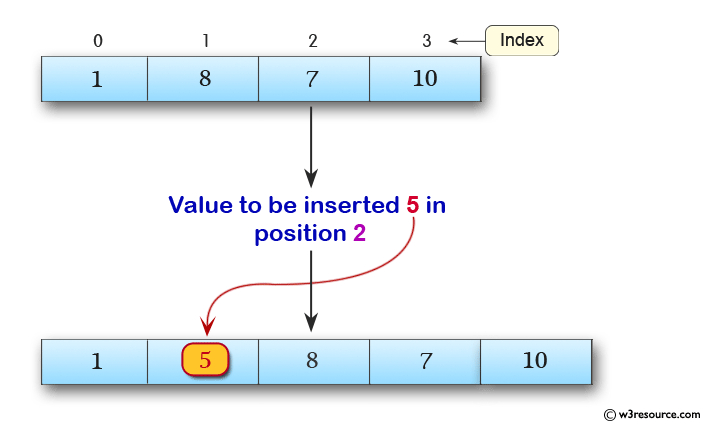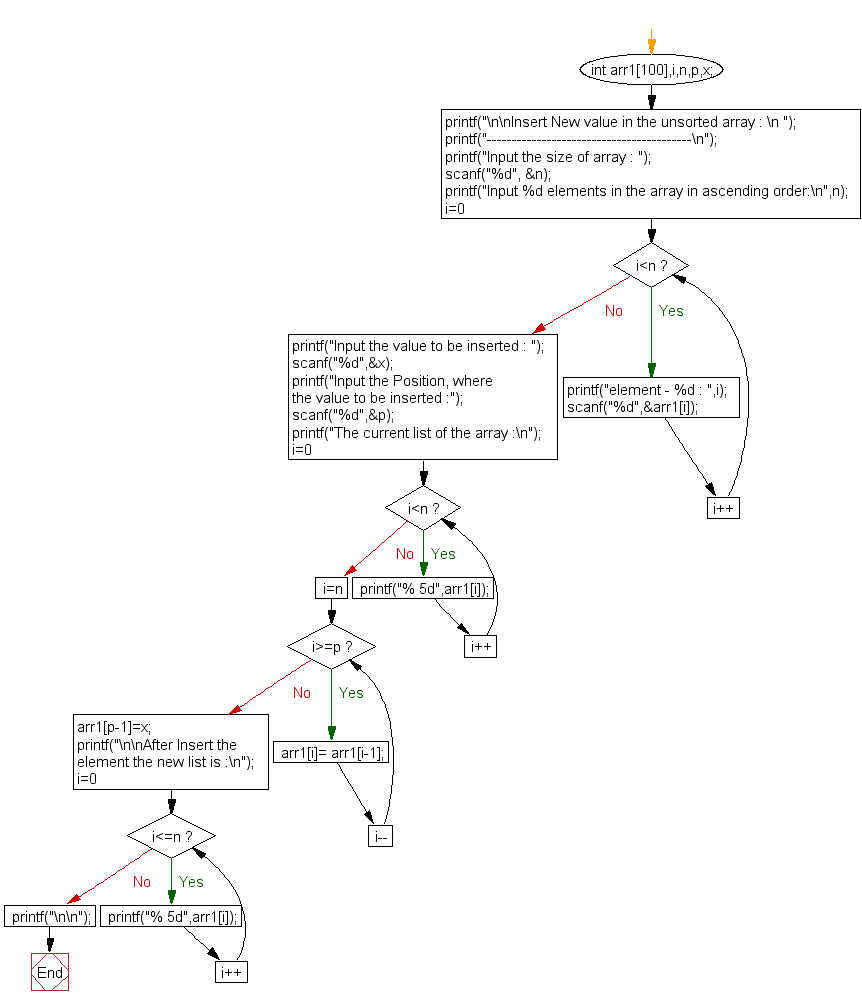﻿ C exercises: Insert New value in the array (unsorted list ) - w3resource# C Exercises: Insert New value in the array (unsorted list )

## C Array: Exercise-14 with Solution

Write a program in C to insert New value in the array (unsorted list ).

Pictorial Presentation:Sample Solution:

C Code:

``````#include <stdio.h>

void main()
{
int arr1,i,n,p,x;

printf("\n\nInsert New value in the unsorted array : \n ");
printf("-----------------------------------------\n");
printf("Input the size of array : ");
scanf("%d", &n);
/* Stored values into the array*/
printf("Input %d elements in the array in ascending order:\n",n);
for(i=0;i<n;i++)
{
printf("element - %d : ",i);
scanf("%d",&arr1[i]);
}

printf("Input the value to be inserted : ");
scanf("%d",&x);
printf("Input the Position, where the value to be inserted :");
scanf("%d",&p);

printf("The current list of the array :\n");
for(i=0;i<n;i++)
printf("% 5d",arr1[i]);
/* Move all data at right side of the array */
for(i=n;i>=p;i--)
arr1[i]= arr1[i-1];
/* insert value at given position */
arr1[p-1]=x;

printf("\n\nAfter Insert the element the new list is :\n");
for(i=0;i<=n;i++)
printf("% 5d",arr1[i]);
printf("\n\n");
}
```
```

Sample Output:

```Insert New value in the unsorted array :
-----------------------------------------
Input the size of array : 4
Input 4 elements in the array in ascending order:
element - 0 : 1
element - 1 : 8
element - 2 : 7
element - 3 : 10
Input the value to be inserted : 5
Input the Position, where the value to be inserted :2
The current list of the array :
1    8    7   10

After Insert the element the new list is :
1    5    8    7   10
```

Flowchart:C Programming Code Editor:

Improve this sample solution and post your code through Disqus.

What is the difficulty level of this exercise?

﻿